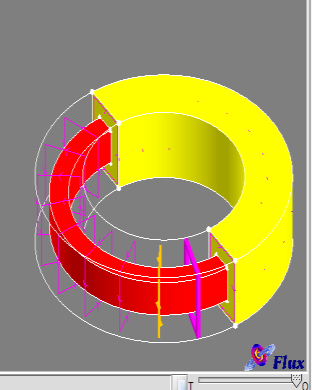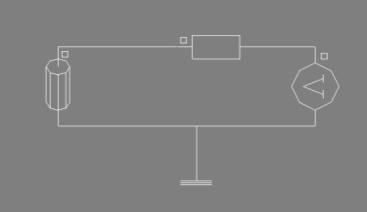# CoilAroundTorus.PFM

## Description

Create a torus with surrounding coil.## Input

• Choose the name of the cut plane
• Select to create or not the torus part
• Select the coordinate system at the center of the torus
• Select the cross section of the torus: Yes=Rectangular/No=Circular
• Define inner diameter of the torus
• Define outer diameter of the torus
• Define torus height (ignore if circular section)
• Define gap angle in torus (in degree)
• Define distance between torus and coil
• Define average coil diameter
• Define fillet radius (if rectangular section)
• Define turn number
• Select Yes=discrete coils/No=continuous turn
• Define coil number (or turn number if continuous)
• Define coil resistance
• Select coil representation: Yes=line/No=volume
• Define angle of coil representation (in degree)
• Select with or without mesh guide (for the coil)

## Output

• Create torus (for magnetic region)
• Create non-meshed coil around the torus
• Create an electrical circuit with voltage and coil